# Great Wall Pizzeria issued 9-year bonds one year ago at a coupon rate of 6.7 percent. If the YTM on these bonds is 8.9...

• Great Wall Pizzeria issued 9-year bonds one year ago at a coupon rate of 6.7 percent. If the YTM on these bonds is 8.9 percent, what is the current bond price?
• LKD Co. has 12 percent coupon bonds with a YTM of 9.9 percent. The current yield on these bonds is 10.4 percent. How many years do these bonds have left until they mature?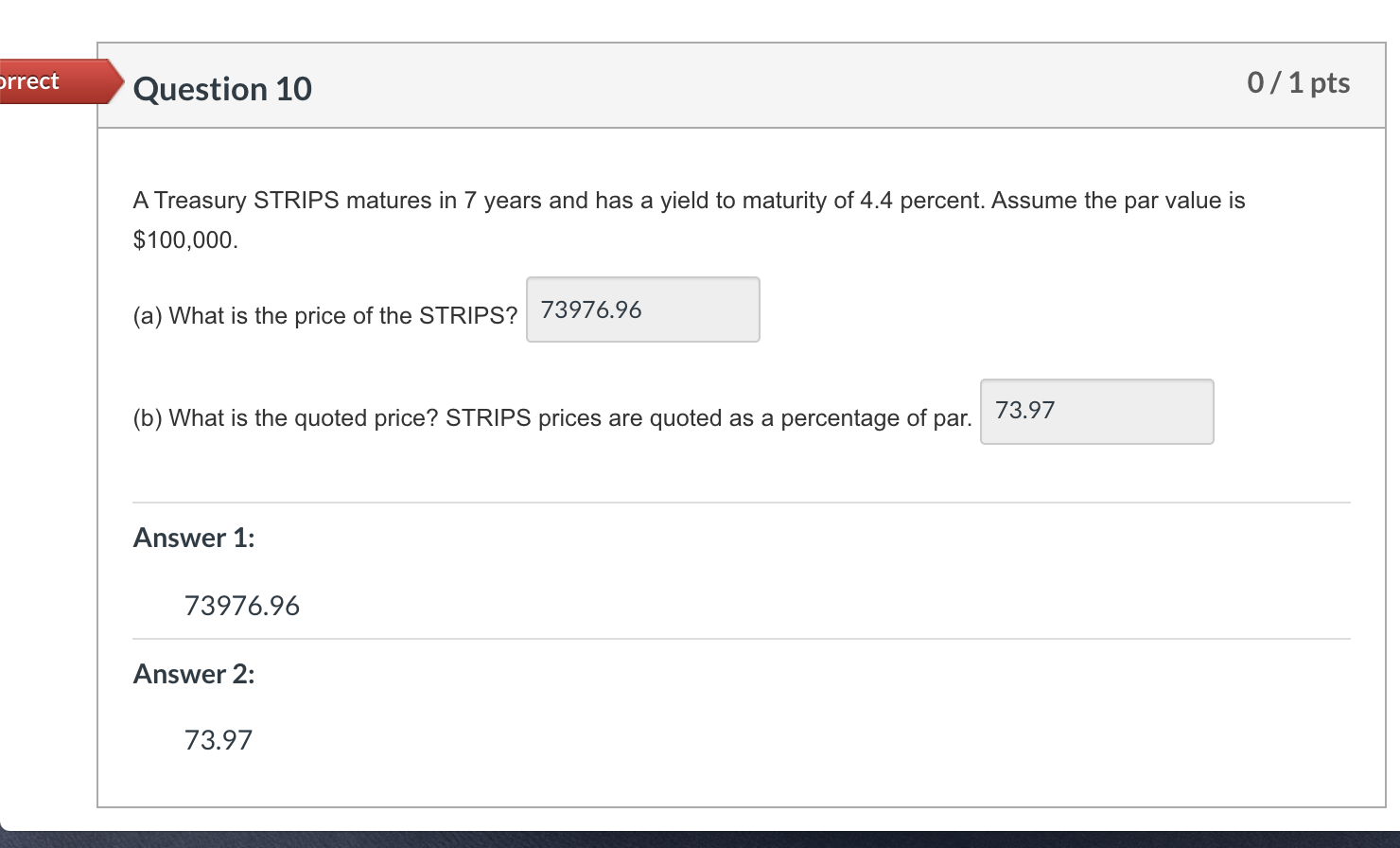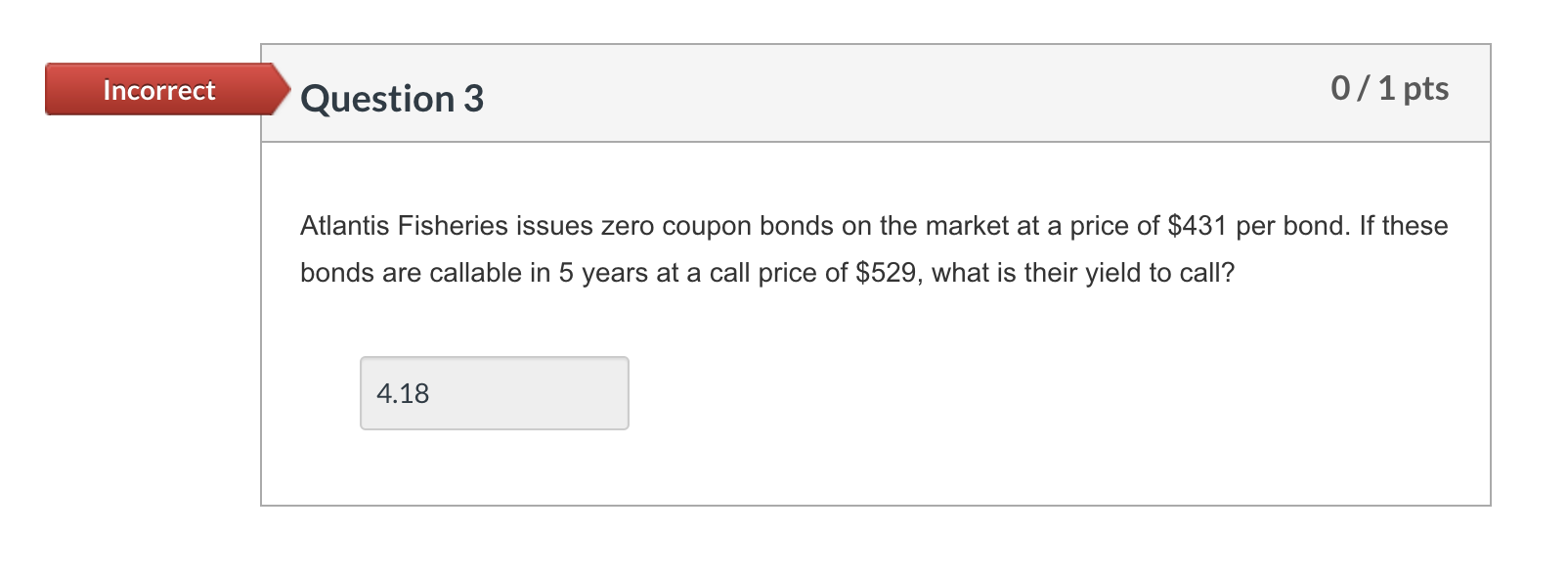orrect Question 10 0/1 pts A Treasury STRIPS matures in 7 years and has a yield to maturity of 4.4 percent. Assume the par value is \$100,000. (a) What is the price of the STRIPS? 73976.96 (b) What is the quoted price? STRIPS prices are quoted as a percentage of par. 73.97 Answer 1: 73976.96 Answer 2: 73.97
Incorrect Question 3 0/1 pts Atlantis Fisheries issues zero coupon bonds on the market at a price of \$431 per bond. If these bonds are callable in 5 years at a call price of \$529, what is their yield to call? 4.18

Using financial calculator

Input: FV= 529

PV = -431

N=5

Solve for I/Y as 4.18

Hence YTC= 4.18% or 0.0418

##### Add Answer of: Great Wall Pizzeria issued 9-year bonds one year ago at a coupon rate of 6.7 percent. If the YTM on these bonds is 8.9...
Similar Homework Help Questions
• ### Atlantis Fisheries issues zero coupon bonds on the market at a price of \$513 per bond. These are callable in 7 year...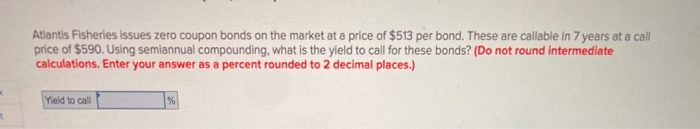Atlantis Fisheries issues zero coupon bonds on the market at a price of \$513 per bond. These are callable in 7 years at a call price of \$590. Using semiannual compounding, what is the yield to call for these bonds? (Do not round intermediate calculations. Enter your answer as a percent rounded to 2 decimal places.) Yield to call

• ### please explain how to calculate in a financial calculator Question 2. MTV Corporation has 7 percent coupon bonds on...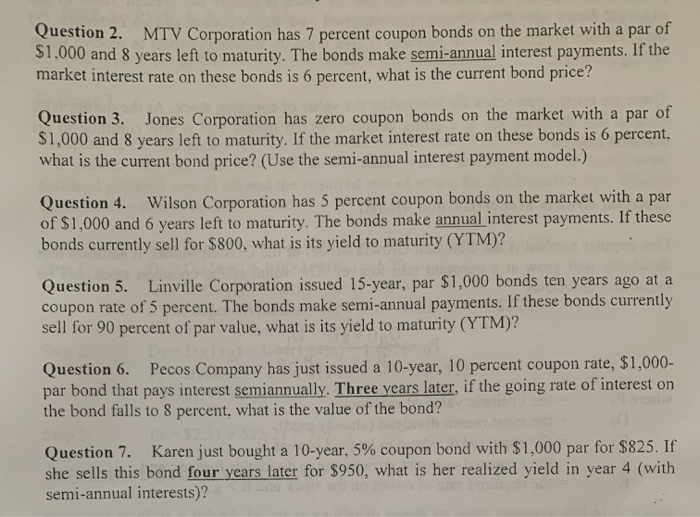please explain how to calculate in a financial calculator Question 2. MTV Corporation has 7 percent coupon bonds on the market with a par of \$1,000 and 8 years left to maturity. The bonds make semi-annual interest payments. If the market interest rate on these bonds is 6 percent, what is the current bond price? Question 3. Jones Corporation has zero coupon bonds on the market with a par of \$1,000 and 8 years left to maturity. If the market...

• ### Ngata Corp. Issued 15-year bonds 2 years ago at a coupon rate of 9.8 percent The...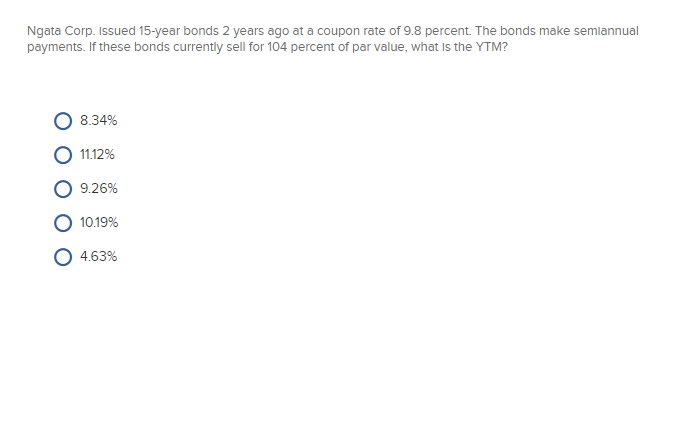Ngata Corp. Issued 15-year bonds 2 years ago at a coupon rate of 9.8 percent The bonds make semiannual payments. If these bonds currently sell for 104 percent of par value, what is the YTM? О 8.34% О 11.12% О 9.26% О 10.19% 0 4.63%

• ### mgm issued 20-year bonds 4 years ago at a coupon rate of 8.5 percent. the bonds make semiannual payments. if these bond...

mgm issued 20-year bonds 4 years ago at a coupon rate of 8.5 percent. the bonds make semiannual payments. if these bonds currently sell for 91.4 percent of par value, what is the YTM?

• ### West Corp. issued 10-year bonds two years ago at a coupon rate of 8.1 percent. The bonds make semiannual payments....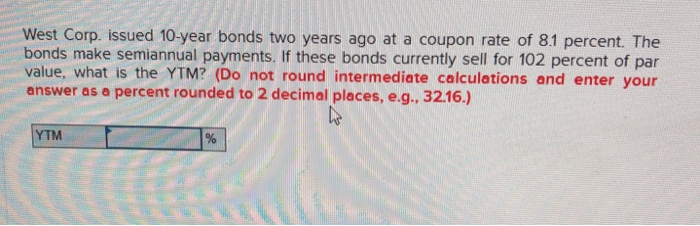West Corp. issued 10-year bonds two years ago at a coupon rate of 8.1 percent. The bonds make semiannual payments. If these bonds currently sell for 102 percent of par value, what is the YTM? (Do not round intermediate calculations and enter your answer as a percent rounded to 2 decimal places, e.g., 32.16.) YTM

• ### please show how to calculate with financial calculator. Question 3. Jones Corporation has zero coupon bonds on the m...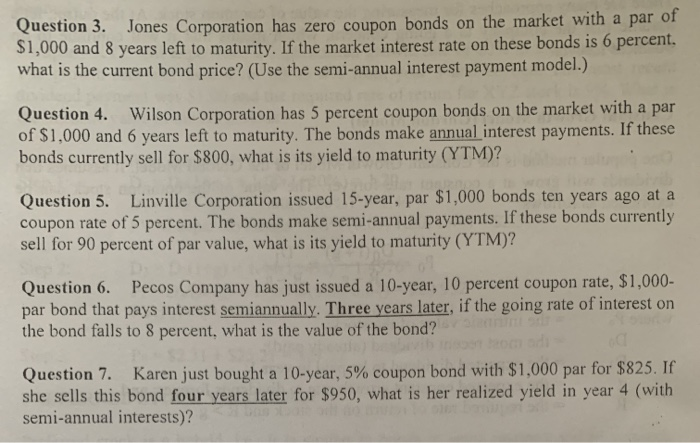please show how to calculate with financial calculator. Question 3. Jones Corporation has zero coupon bonds on the market with a par of s1,000 and 8 years left to maturity. If the market interest rate on these bonds is 6 percent what is the current bond price? (Use the semi-annual interest payment model.) Question 4. Wilson Corporation has 5 percent coupon bonds on the market with a par of \$1,000 and 6 years left to maturity. The bonds make annual...

• ### (a) Is the yield to maturity (YTM) on a bond the same thing as the required...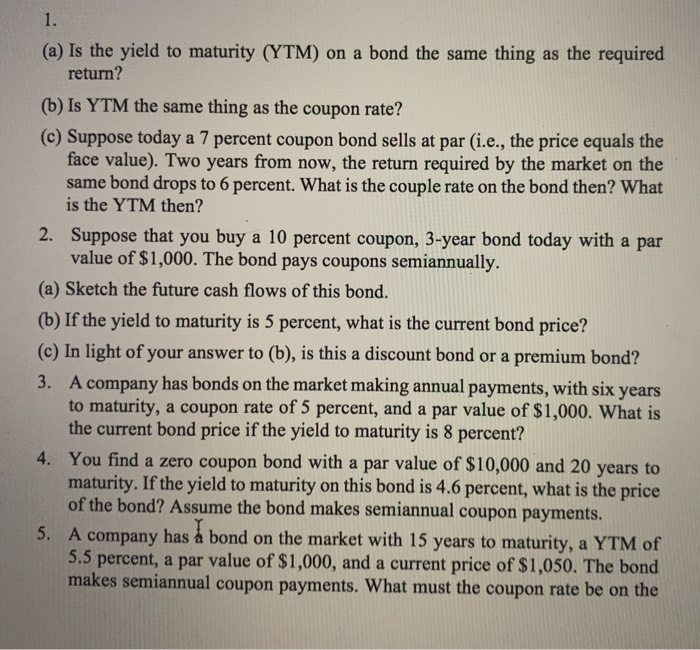(a) Is the yield to maturity (YTM) on a bond the same thing as the required return? (b) Is YTM the same thing as the coupon rate? (c) Suppose today a 7 percent coupon bond sells at par (i.e., the price equals the face value). Two years from now, the return required by the market on the same bond drops to 6 percent. What is the couple rate on the bond then? What is the YTM then? 2. Suppose that...

• ### A Treasury STRIPS matures in 8 years and has a yield to maturity of 5.4 percent. Assume the par value is \$100,000....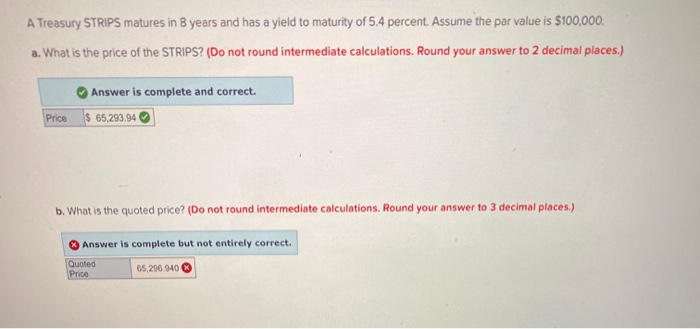A Treasury STRIPS matures in 8 years and has a yield to maturity of 5.4 percent. Assume the par value is \$100,000. a. What is the price of the STRIPS? (Do not round intermediate calculations. Round your answer to 2 decimal places.) Answer is complete and correct. \$ 65,293.94 Price b. What is the quoted price? (Do not round intermediate calculations. Round your answer to 3 decimal places.) Answer is complete but not entirely correct. Quoted Price 65,206.940

• ### Please include formulas Problem 6-6 Bond Prices [LO 2] App Store Co. issued 13-year bonds one year ago at a coupon rate...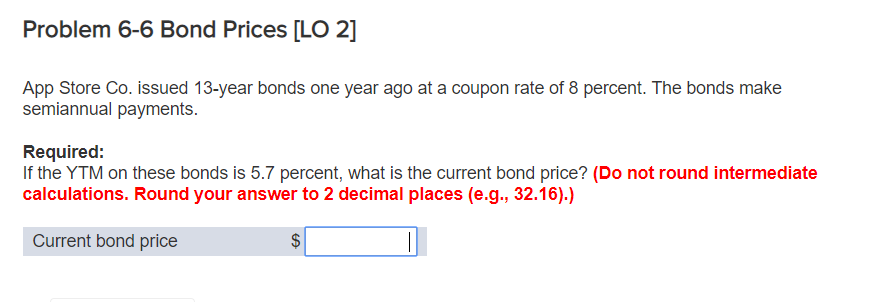Please include formulas Problem 6-6 Bond Prices [LO 2] App Store Co. issued 13-year bonds one year ago at a coupon rate of 8 percent. The bonds make semiannual payments. Required: If the YTM on these bonds is 5.7 percent, what is the current bond price? (Do not round intermediate calculations. Round your answer to 2 decimal places (e.g., 32.16).) Current bond price

• ### 7. Valuing semiannual coupon bonds Bonds often pay a coupon twice a year. For the valuation of bonds that make semiannu...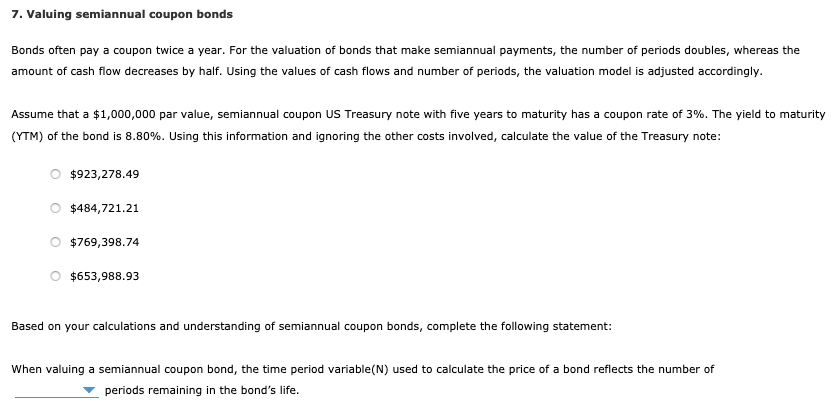7. Valuing semiannual coupon bonds Bonds often pay a coupon twice a year. For the valuation of bonds that make semiannual payments, the number of periods doubles, whereas the amount of cash flow decreases by half. Using the values of cash flows and number of periods, the valuation model is adjusted accordingly. Assume that a \$1,000,000 par value, semiannual coupon US Treasury note with five years to maturity has a coupon rate of 3%. The yield to maturity (YTM) of...

Need Online Homework Help?